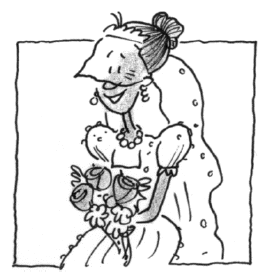### Home > CALC > Chapter 7 > Lesson 7.2.3 > Problem7-87

7-87.

THE WEDDING CAKE, Part Two

Kiki is still trying to decide on her wedding cake. She decided to keep the same shape and size but instead have $8$ layers. Therefore, the diameters of the layers would be $16$, $14$, $12$, $10$, $8$, $6$, $4$, and $2$ inches. Each layer for this cake would only be $2$ inches tall.

1. Set up a Riemann sum to evaluate the volume of the cake.

A Riemann sum does NOT have to be written in sigma notation.
Your setup should be concise and preferably written as a single equation.

2. Find the volume of this latest design.

$V = 2π(8^2 + 7^2 + ... )\operatorname{in}3$